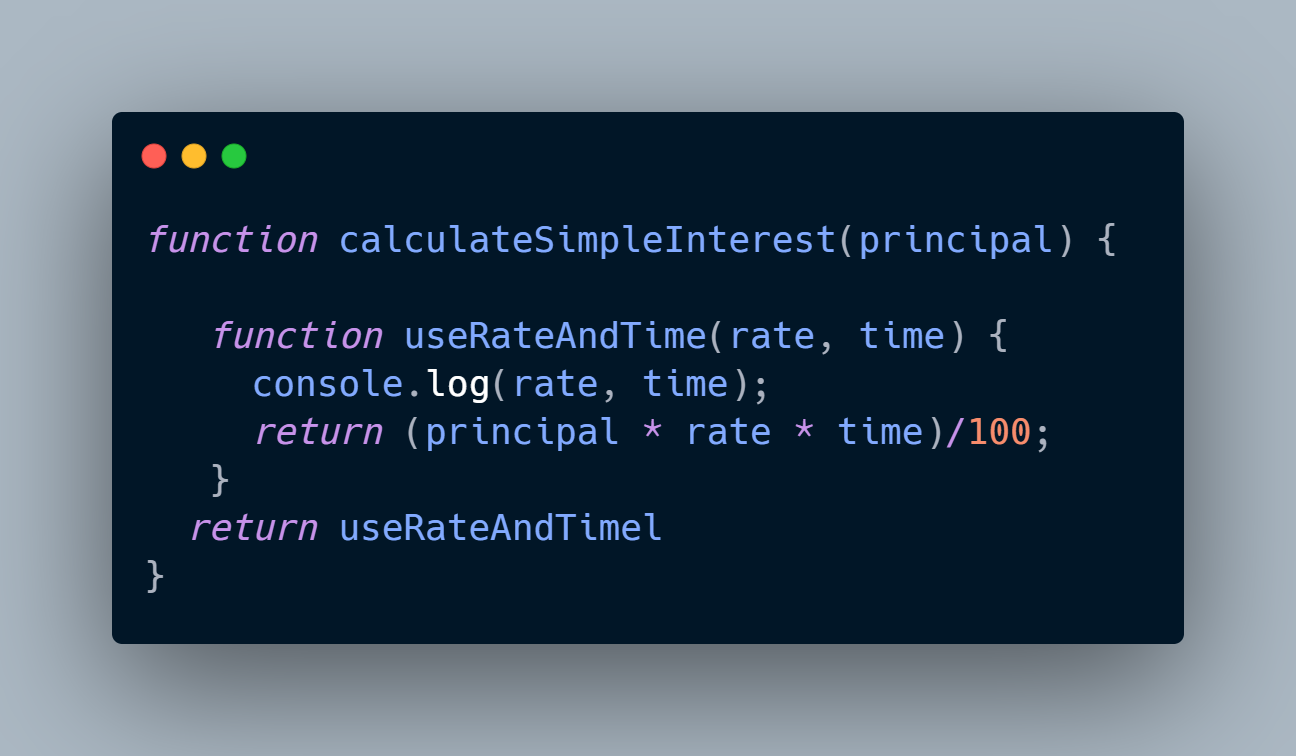Categories

# Demystifying Closures in JavaScript

Hey guys 👋🏻,

✔ What are Closures ?
✔ Code Example with explanation.
✔ Conclusion

## What are Closures ?

Before understanding what a closure is, let us revisit the concept of functions. From what we know about functions in JavaScript is that every function in JavaScript has a reference to its outer lexical environment.

This means that it registers the outer lexical environment and the variables present in there and it remembers the values of these variables.

=> This also means that the reference that gets setup enables the code inside the inner function to see variables declared outside the inner function, regardless of when and where the function was called.

So let us see an example,## Explanation

In the above code, we have a `calculateSimpleInterest` function that takes on a `principal` value inside which we have function `useRateAndTime` that takes on `rate` and `time` and computes the simple interest for us. At the bottom of the `calculateSimpleInterest` function, we return the `useRateAndTime` function.

So for our `calculateSimpleInterest` function, `useRateAndTime` forms a closure with the lexical environment of the execution context which gets created when `calculateSimpleInterest` function is invoked, closing over the variables defined inside the outer function (if any).

## Let us see the usage

With the invocation of `calculateSimpleInterest` function using a value of 10000 as `principal`, a new function `useRateAndTime` gets created and in it the value of `principal` gets locked and is returned. Now we have the `useRateAndTimeFn` constant in which we have the returned function having the `principal` locked in. So we call the inner function now by passing the value of `rate` and `time`. Lastly we store the returned value in a variable with the name of `result`.

## Conclusion

✔ Use closures if you want a function to always have access to a private piece of state.
✔ In JavaScript, if you declare a function within another function, then the local variables of the outer function can remain accessible even after returning from the outer function.